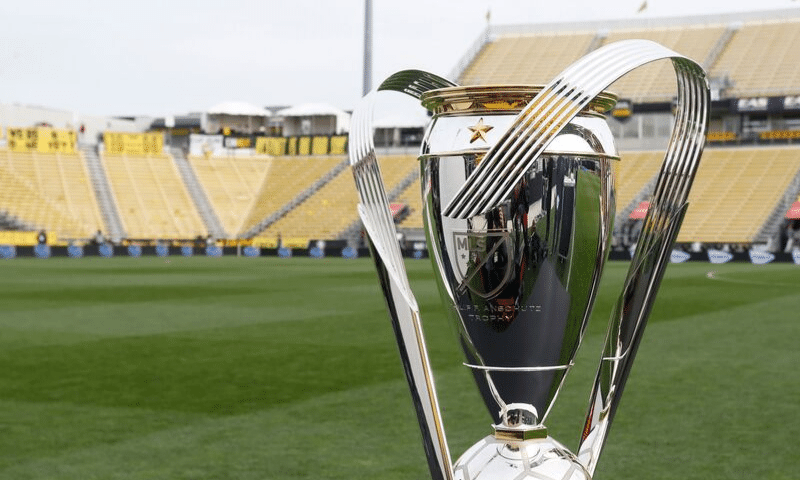Intro text, can be displayed through an additional field

## How Many Mls Is 1/3 Cup

When it comes to cooking and baking, precise measurements are crucial for achieving the perfect results. However, converting measurements can sometimes be a bit confusing, especially when dealing with different units of measurement. If you find yourself wondering how many milliliters (mls) are in 1/3 cup, this article will provide you with a detailed answer. So, let's dive in!

### The Conversion: Cups to Milliliters

Before we determine how many milliliters are in 1/3 cup, we need to understand the basic conversion from cups to milliliters. The conversion factor for this conversion is as follows:

1 cup = 236.59 milliliters (mls)

With this conversion factor in mind, we can now calculate how many milliliters are in 1/3 cup:

#### Calculating 1/3 Cup in Milliliters

To calculate 1/3 cup in milliliters, we can use the following equation:

1/3 cup = (1/3) * 236.59 mls

Now, let's do the math:

1/3 cup = (1/3) * 236.59

1/3 cup = 78.8633 mls

Therefore, 1/3 cup is approximately equal to 78.86 milliliters (mls).

### Why Is Accurate Measurement Important?

Accurate measurement is crucial in cooking and baking for a variety of reasons:

• Consistent Results: Accurate measurements ensure that you achieve consistent results every time you prepare a recipe.
• Recipe Ratios: Recipes often rely on specific ingredient ratios for taste, texture, and overall success. Incorrect measurements can throw off these ratios and affect the final outcome.
• Chemical Reactions: In baking, precise measurements are particularly important because many ingredients, such as leavening agents, rely on chemical reactions to create the desired texture and rise.

#### Q: Can I Use a Different Cup Size for the Conversion?

A: The conversion factor provided is specifically for the standard U.S. cup, which is equivalent to 236.59 milliliters. If you are using a different cup size, such as the metric cup (250 milliliters) or the UK cup (284 milliliters), the conversion will be slightly different.

#### Q: How Can I Measure 1/3 Cup Without a Measuring Cup?

A: If you do not have a measuring cup, there are alternative ways to measure 1/3 cup. You can use a kitchen scale to weigh the ingredients or use measuring spoons. One-third cup is equivalent to approximately 5 tablespoons plus 1 teaspoon.

#### Q: Is It Important to Level the Cup When Measuring?

A: Yes, it is essential to level the cup when measuring dry ingredients. Leveling ensures that you have the precise amount required in the recipe. To level, use a straight-edged utensil (like a knife or spatula) to remove any excess ingredient from the top of the cup.

### Conclusion

Knowing how to convert measurements is an essential skill in the kitchen. When it comes to determining how many milliliters are in 1/3 cup, the conversion factor of 236.59 milliliters per cup is used. Therefore, 1/3 cup is approximately equal to 78.86 milliliters (mls). Accurate measurement is crucial for achieving consistent results and maintaining proper ingredient ratios. So, the next time you need to measure 1/3 cup, you can confidently convert it to milliliters!

## Related video of How Many Mls Is 1 3 Cup

Ctrl
Enter
Noticed oshYwhat?
Highlight text and click Ctrl+Enter
We are in
Recipe » Press » How Many Mls Is 1 3 Cup